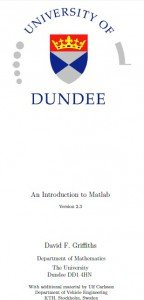# Matlab Notes

An Introduction to Matlab By David F. Griths
Department of Mathematics, The University of DundeeMatlab Notes includes the following

1. MATLAB
2. Starting Up
3. Matlab as a Calculator
4. Numbers & Formats
5. Variables
6. Suppressing output
7. Built{In Functions
8. Vectors
9. Keeping a record
10. Plotting Elementary Functions
11. Keyboard Accelerators
12. Copying to and from Word and other applications
13. Script Files
14. Products, Division & Powers of Vectors
15. Examples in Plotting
16. Matrices|Two{Dimensional Arrays
17. Systems of Linear Equations
18. Characters, Strings and Text
19. Loops
20. Logicals
21. Function m-files
22. Further Built{in Functions
23. Plotting Surfaces
24. Timing
25. On-line Documentation
26. Reading and Writing Data Files
27. Command Summary

1.Fahad on said:
•UBAIDAHMAD on said: